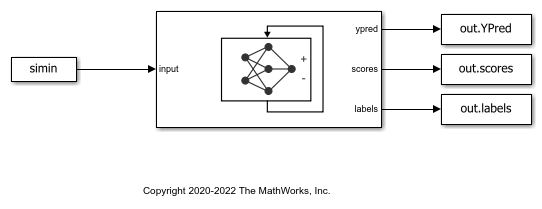# Classify and Update Network State in Simulink

This example shows how to classify data for a trained recurrent neural network in Simulink® by using the `Stateful Classify` block. This example uses a pretrained long short-term memory (LSTM) network.

Load `JapaneseVowelsNet`, a pretrained long short-term memory (LSTM) network trained on the Japanese Vowels data set as described in  and . This network was trained on the sequences sorted by sequence length with a mini-batch size of 27.

```load JapaneseVowelsNet ```

View the network architecture.

```analyzeNetwork(net); ```

Load the Japanese Vowels test data. `XTest` is a cell array containing 370 sequences of dimension 12 of varying length. `TTest` is a categorical vector of labels "1","2",..."9", which correspond to the nine speakers.

Create a timetable array `simin` with time-stamped rows and repeated copies of `X`.

```[XTest,TTest] = japaneseVowelsTestData; X = XTest{94}; numTimeSteps = size(X,2); simin = timetable(repmat(X,1,4)','TimeStep',seconds(0.2)); ```

### Simulink Model for Classifying Data

The Simulink model for classifying data contains a `Stateful Classify` block to predict the labels and `From Workspace` block to load the input data sequence over the time steps.

To reset the state of recurrent neural network to its initial state during simulation, place the `Stateful Classify` block inside a `Resettable Subsystem` and use the `Reset` control signal as trigger.

```open_system('StatefulClassifyExample'); ```### Configure Model for Simulation

Set the model configuration parameters for the `Stateful Classify` block.

```set_param('StatefulClassifyExample/Stateful Classify','NetworkFilePath','JapaneseVowelsNet.mat'); set_param('StatefulClassifyExample','SimulationMode','Normal'); ```

### Run the Simulation

To compute responses for the `JapaneseVowelsNet` network, run the simulation. The prediction labels are saved in the MATLAB® workspace.

```out = sim('StatefulClassifyExample'); ```

Plot the predicted labels in a stair plot. The plot shows how the predictions change between time steps.

```labels = squeeze(out.YPred.Data(1:numTimeSteps,1)); figure stairs(labels, '-o') xlim([1 numTimeSteps]) xlabel("Time Step") ylabel("Predicted Class") title("Classification Over Time Steps") ```Compare the predictions with the true label. Plot a horizontal line showing the true label of the observation.

```trueLabel = double(TTest(94)); hold on line([1 numTimeSteps],[trueLabel trueLabel], ... 'Color','red', ... 'LineStyle','--') legend(["Prediction" "True Label"]) axis([1 numTimeSteps+1 0 9]); ```M. Kudo, J. Toyama, and M. Shimbo. "Multidimensional Curve Classification Using Passing-Through Regions." Pattern Recognition Letters. Vol. 20, No. 11–13, pages 1103–1111.

 UCI Machine Learning Repository: Japanese Vowels Dataset. https://archive.ics.uci.edu/ml/datasets/Japanese+Vowels